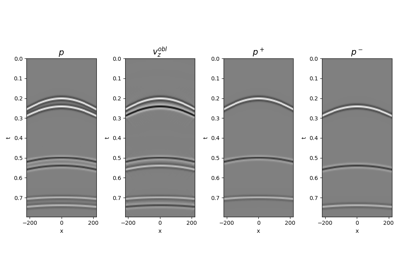# pylops.waveeqprocessing.PressureToVelocity#

pylops.waveeqprocessing.PressureToVelocity(nt, nr, dt, dr, rho, vel, nffts=(None, None, None), critical=100.0, ntaper=10, topressure=False, backend='numpy', dtype='complex128', name='P')[source]#

Pressure to Vertical velocity conversion.

Apply conversion from pressure to vertical velocity seismic wavefield (or vertical velocity to pressure). The input model and data required by the operator should be created by flattening the a wavefield of size $$(\lbrack n_{r_y} \times n_{r_x} \times n_t \rbrack$$.

Parameters
ntint

Number of samples along the time axis

nr

Number of samples along the receiver axis (or axes)

dtfloat

Sampling along the time axis

dr

rhofloat

Density $$\rho$$ along the receiver array (must be constant)

velfloat

Velocity $$c$$ along the receiver array (must be constant)

nfftstuple, optional

Number of samples along the wavenumber and frequency axes

criticalfloat, optional

Percentage of angles to retain in obliquity factor. For example, if critical=100 only angles below the critical angle $$\sqrt{k_y^2 + k_x^2} < \frac{\omega}{c}$$ will be retained

ntaperfloat, optional

Number of samples of taper applied to obliquity factor around critical angle

topressurebool, optional

Perform conversion from particle velocity to pressure (True) or from pressure to particle velocity (False)

backendstr, optional

Backend used for creation of obliquity factor operator (numpy or cupy)

dtypestr, optional

Type of elements in input array.

namestr, optional

New in version 2.0.0.

Name of operator (to be used by pylops.utils.describe.describe)

Returns
Coppylops.LinearOperator

Pressure to particle velocity (or particle velocity to pressure) conversion operator

UpDownComposition2D

2D Wavefield composition

UpDownComposition3D

3D Wavefield composition

WavefieldDecomposition

Wavefield decomposition

Notes

A pressure wavefield $$p(x, t)$$ can be converted into an equivalent vertical particle velocity wavefield $$v_z(x, t)$$ by applying the following frequency-wavenumber dependant scaling :

$\hat{v}_z(k_x, \omega) = \frac{k_z}{\omega \rho} \hat{p}(k_x, \omega)$

where the vertical wavenumber $$k_z$$ is defined as $$k_z=\sqrt{\frac{\omega^2}{c^2} - k_x^2}$$.

Similarly a vertical particle velocity can be converted into an equivalent pressure wavefield by applying the following frequency-wavenumber dependant scaling :

$\hat{p}(k_x, \omega) = \frac{\omega \rho}{k_z} \hat{v}_z(k_x, \omega)$

For 3-dimensional applications the only difference is represented by the vertical wavenumber $$k_z$$, which is defined as $$k_z=\sqrt{\frac{\omega^2}{c^2} - k_x^2 - k_y^2}$$.

In both cases, this operator is implemented as a concatanation of a 2 or 3-dimensional forward FFT (pylops.signalprocessing.FFT2 or pylops.signalprocessing.FFTN), a weighting matrix implemented via pylops.basicprocessing.Diagonal, and 2 or 3-dimensional inverse FFT.

1(1,2)

Wapenaar, K. “Reciprocity properties of one-way propagators”, Geophysics, vol. 63, pp. 1795-1798. 1998.

## Examples using pylops.waveeqprocessing.PressureToVelocity#14. Seismic wavefield decomposition

14. Seismic wavefield decomposition